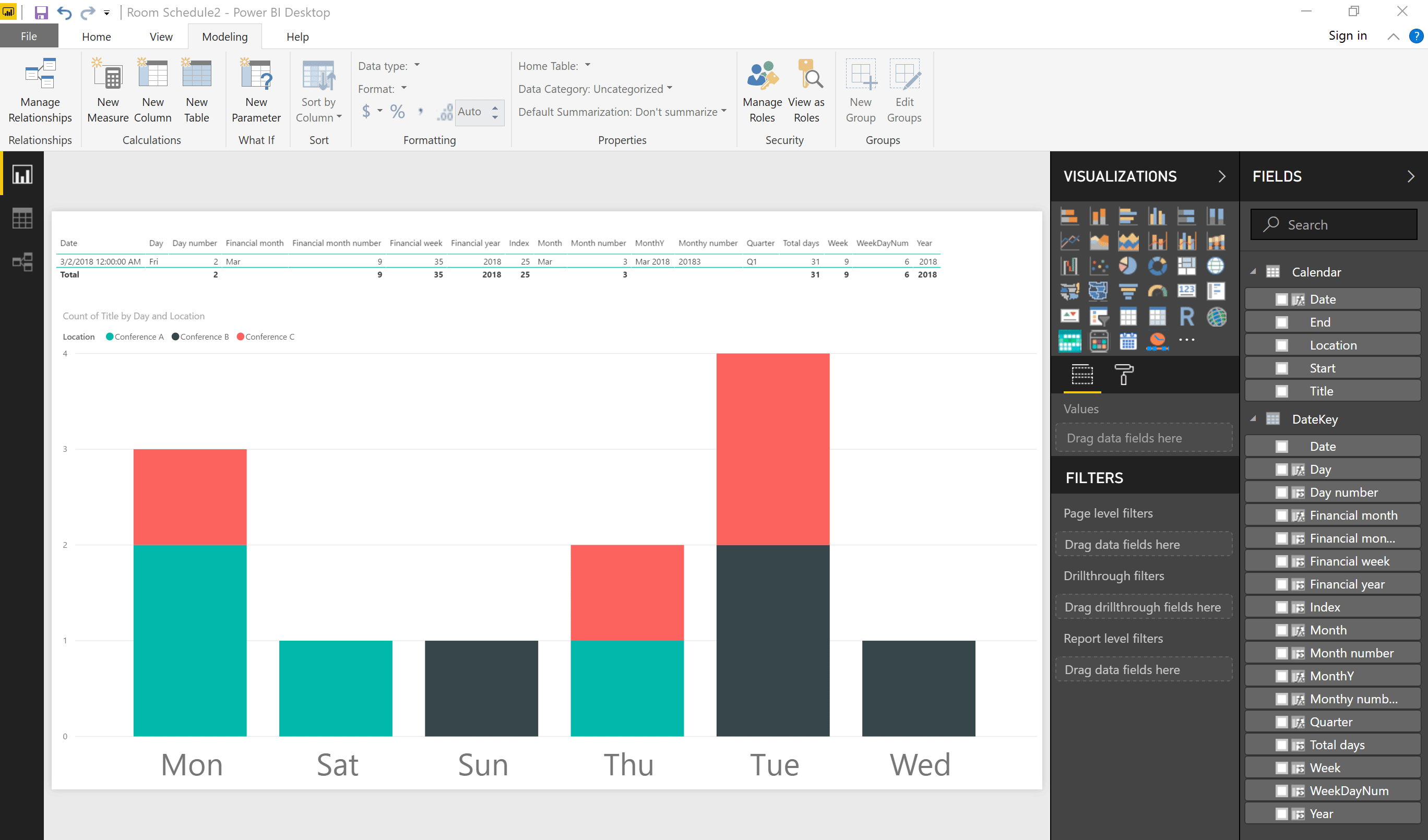# How to create a powerful date table or DateKey in Power BI

DateKeys are essential for relative time measures. In “manage relationships” you tie the ‘DateKey'[Date] to a date field in each of your data sources. Giles Walker shared an excellent solution for a robust DateKey that includes measurements and calculations you’re sure to find useful. Here’s that same solution I’ve modified and expanded to be as useful as possible.

## Creating the Table

1. Have at least one data source loaded into your report that uses a date field to which you’ll be tying.
2. Click “New Table” from the modeling tab3. Enter the following formula in the formula bar, replacing ‘Calendar'[Start] and ‘Calendar'[End] with your own column names from your data source. It could be the same column for both if you just have one measure like ‘Sales'[Date].
DateKey = CALENDAR(min(‘Calendar'[Start]),max(‘Calendar'[End]))

## Creating columns

Now you have a DateKey ranging from your earliest date to your latest in your data. Add the following columns as you wish:

1. Modeling –> New Column
2. Copy and paste each formula below and click the “checkmark” to save
3. Repeat

### Result

`DateKey = CALENDAR(min('Calendar'[Start]),max('Calendar'[End]))`
3/2/2018
`Day = FORMAT(DateKey[Date],"ddd")`
Fri
`Day number = DAY(DateKey[Date])`
2
`Financial month = FORMAT(DateKey[Date],"MMM")`
Mar
`Financial month number = IF((DateKey[Month number]-6)<=0,DateKey[Month number]+6,DateKey[Month number]-6)`
9
`Financial week = IF(DateKey[Month number]>6,DateKey[Week]-26,DateKey[Week]+26)`
35
`Financial year = IF(DateKey[Month number]>6,DateKey[Year]+1,DateKey[Year])`
2018
`Index = CALCULATE(COUNT(DateKey[Date]),ALL(DateKey[Date]),FILTER(DateKey,DateKey[Date]<=EARLIER(DateKey[Date])))`
21
`Month = FORMAT(DateKey[Date],"MMM")`
Mar
`Month number = MONTH(DateKey[Date])`
3
`MonthY = FORMAT(DateKey[Date],"MMM")&" " &DateKey[Year]`
Mar-18
`Monthy number = DateKey[Year]&FORMAT(DateKey[Month number],"mm")`
201803
`Quarter = "Q" & ROUNDUP(MONTH(DateKey[Date])/3,0)`
Q1
`Total days = DAY(DATE(DateKey[Year],DateKey[Month number]+1,1)-1)`
31
`Week = WEEKNUM(DateKey[Date],1)`
9
`WeekDayNum = WEEKDAY(DateKey[Date],1)`
6
`Year = YEAR(DateKey[Date])`
2018
1. You’ll want to be sure to sort the following columns so days of the week, months, etc. show up in the correct order in your visuals. Click the first column, “Sort by Column” on the modeling tab and click the sorting column.
1. Sort Day by WeekDayNum
2. Sort Month by Month number
3. Sort MonthY by Monthy number## Creating measures

1. Modeling –> New measure
2. Copy and paste each row below and click the “checkmark” to save
3. Repeat
 `Current financial week = IF(WEEKNUM(NOW())-26<=0,WEEKNUM(NOW())+26,WEEKNUM(NOW())-26)` `Current financial month = IF(MONTH(NOW())-6<=0,MONTH(NOW())+6,MONTH(NOW())-6)` `Today = DATE(YEAR(NOW()),MONTH(NOW()),DAY(NOW()))`

## Creating columns for use as filters (i.e. Show only if value=1)

1. Modeling –> New Column
2. Copy and paste each row below and click the “checkmark” to save
3. Repeat
 `+/- 1 months = IF(AND(DateKey[Financial month number]>=[Current financial month]-1,DateKey[Financial month number]<=[Current financial month]),1,0)` `Last 30 day = IF(AND(DateKey[Date]>=[Today]-30,DateKey[Date]<=[Today]),1,0)` `Last week + two weeks = IF(AND(DateKey[Financial week]>=[Current financial week]-1,DateKey[Financial week]<=[Current financial week]+2),1,0)` `Last 14 days = IF(AND(DateKey[Date]>=[Today]-14,DateKey[Date]<=[Today]),1,0)` `+/- 30 days = IF(AND(DateKey[Date]>=[Today]-30,DateKey[Date]<=[Today]+30),1,0)`

See more ideas for date formulas and calculations in my recent post, Use Power BI to create a dynamic/live meeting room schedule. It includes “duration” (minutes or hours), “currently happening” (yes/no) and “in the future” (yes/no) columns.

This site uses Akismet to reduce spam. Learn how your comment data is processed.